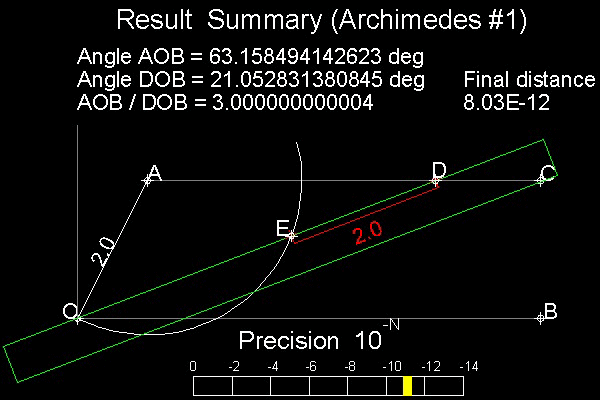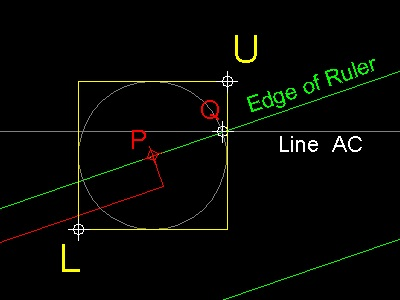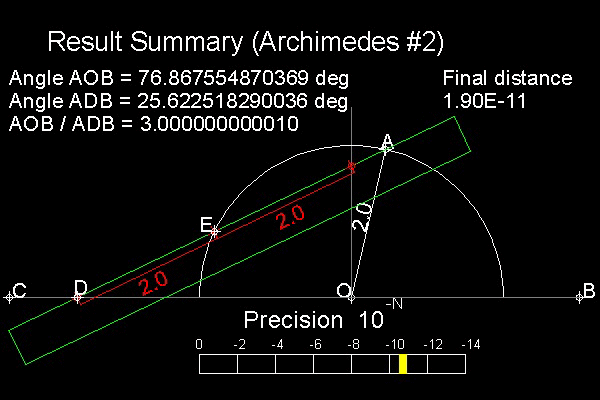History of the Problems
Go to   Fun_Math Content Table   Trisecting an Angle   Classical Solutions

### Classical solutions by Insertion (Neusis or Verging) method

#### 1. Archimedes' Insertion Method #1

Archimedes' Solution is shown in the figure shown below.Angle AOB is to be trisected. Draw a line AC parallel to B

Draw a circle with radius = OA with center at A.

Bring a straight-edge marked with ED = OA

Move this ruler such that it passes through point O, "E" on the circle , and "D" on the line AC.

When it is done, angle DOB is 1/3 of angle AOB

Why ? Hint: AO = AE = ED---> Trangles AOE & AED are isosceles.
************************* trisect_Archimedes_1.dwg *****************#### How to find the trisecting point "D"

The straight edge (in green color) is allowed to move with two constraints:

1. It passes through point "O"

2. Point "E" is always on the circle

Then the problem is reduced to make the point "D" as close as possible to the line AC

This is done by checking the distance from point "D" to line AC and keep zooming to point "D". Without zooming the best human eyes can do is about one hundredth of line length OA. By continuous zooming centered on the point "D" , the distance, shown in the precision bar in yellow can be pushed to 10e-12(10 raised to the power of -12) which is close to the limit of program's precision.
*************** auto_zoom.dwg **************
You can see the process in animation.

To create this drawing and animation:
Then from command line, type neusis_1

#### 2. Pappus' Insertion method

Pappus' Solution is shown in the figure shown below.Angle AOB is to be trisected. Draw a line AC parallel to B

Draw a line AF perpendicular to OB, F on the line OB.

Bring a straight-edge marked with ED = 2 * OA

Move this ruler such that it passes through point O, "E" on the line AF , and "D" on the line AC.

When it is done, angle DOB is 1/3 of angle AOB

Why ? Hint: Since angle EAD is 90 degrees, 3 points A,D, E is on the circle with diamter ED.

So the center of the circle and these 3 points make 2 isosceles triangles.
************************* trisect_Pappus_1.dwg *******************

#### How to find the trisecting point "D"

The straight edge (in green color) is allowed to move with two constraints:

1. It passes through point "O"

2. Point "E" is always on the line AF

Then the problem is reduced to make the point "D" as close as possible to the line AC

This is done by checking the distance from point "D" to line AC and keep zooming to point "D". Without zooming the best human eyes can do is about one hundredth of line length OA. By continuous zooming centered on the point "D" , the distance, shown in the precision bar in yellow can be pushed to 10e-12(10 raised to the power of -12) which is close to the limit of program's precision.

You can see the process in animation.animation

To create this drawing and animation:
Then from command line, type neusis_2

#### 3. Archimedes' Insertion Method #2

Archimedes' Solution is shown in the figure shown below.Angle AOB is to be trisected. Draw a line AC parallel to B

Draw a circle with radius = OA with center at O. Additionmal marker beyond "E" is for reference purpose.

Bring a straight-edge marked with ED = OA

Move this ruler such that it passes through point O, "E" on the circle , and "D" on the line AC.

When it is done, angle DOB is 1/3 of angle AOB

Why ? Hint: AO = EO = ED---> Trangles OAE & EDO are isosceles.

Compare this with the above 2 methods. You will see the similarity.
************************* trisect_Archimedes_2.dwg *******************

#### How to find the trisecting point "D"

The straight edge (in green color) is allowed to move with two constraints:

1. It passes through point "A"

2. Point "E" is always on the circle

Then the problem is reduced to make the point "D" as close as possible to the line BC

This is done by checking the distance from point "D" to line BC and keep zooming to point "D". Without zooming the best human eyes can do is about one hundredth of line length OA. By continuous zooming centered on the point "D" , the distance, shown in the precision bar in yellow can be pushed to 10e-12(10 raised to the power of -12) which is close to the limit of program's precision.

You can see the process in animation.animation

To create this drawing and animation:
Then from command line, type neusis_3

#### References

1. Yates,Robert Carl : "The Trisection problem"

Go to   Fun_Math Content Table   Trisecting an Angle   Classical Solutions

All questions/suggestions should be sent to Takaya Iwamoto

Last Updated Nov 22, 2006